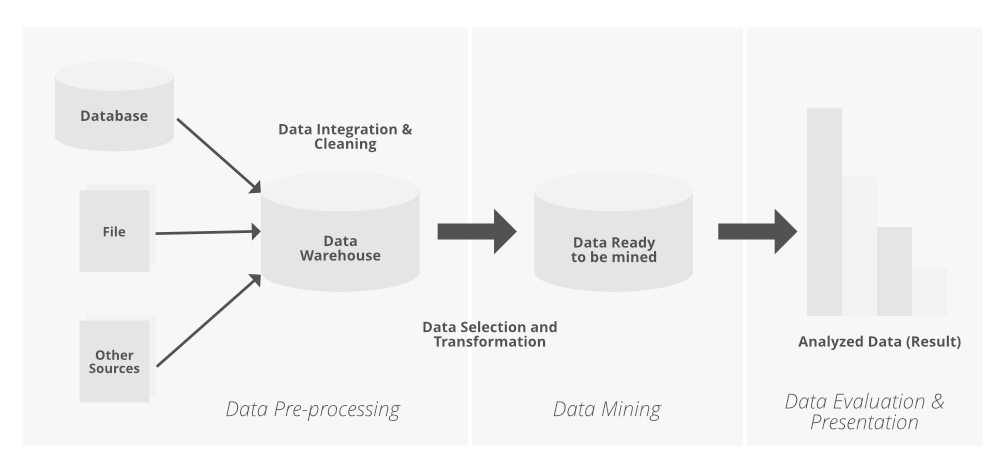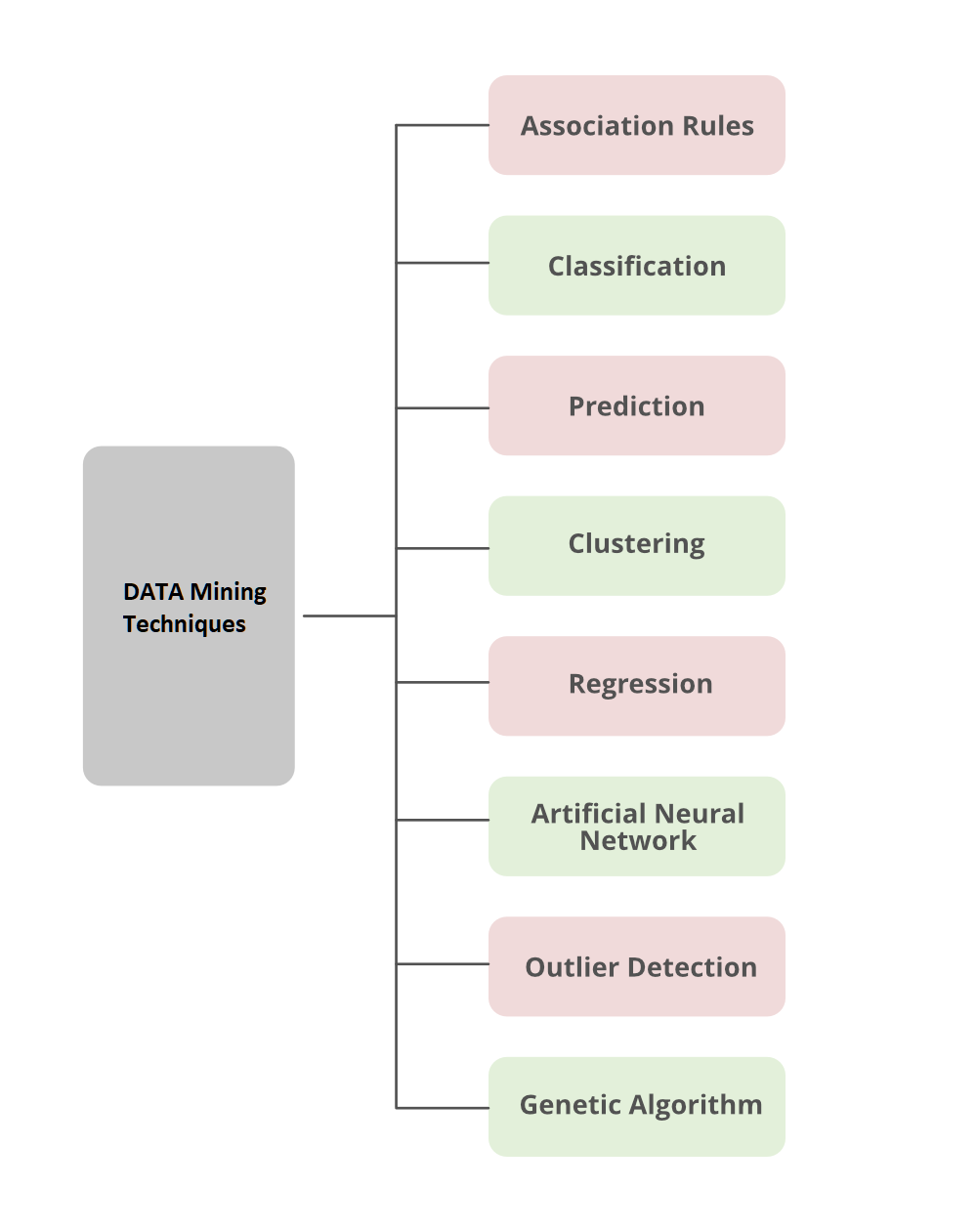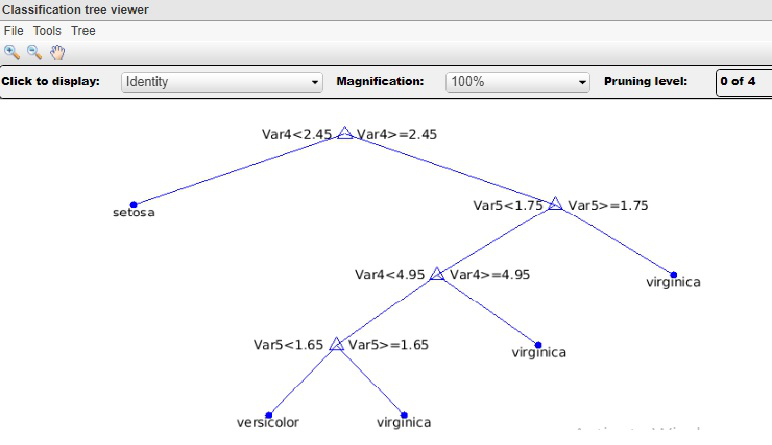# Data Mining Techniques

• Difficulty Level : Medium
• Last Updated : 01 Jun, 2021

Data mining refers to extracting or mining knowledge from large amounts of data. In other words, Data mining is the science, art, and technology of discovering large and complex bodies of data in order to discover useful patterns. Theoreticians and practitioners are continually seeking improved techniques to make the process more efficient, cost-effective, and accurate. Many other terms carry a similar or slightly different meaning to data mining such as knowledge mining from data, knowledge extraction, data/pattern analysis data dredging.

Data mining treats as a synonym for another popularly used term, Knowledge Discovery from Data, or KDD. In others view data mining as simply an essential step in the process of knowledge discovery, in which intelligent methods are applied in order to extract data patterns.

Gregory Piatetsky-Shapiro coined the term “Knowledge Discovery in Databases” in 1989. However, the term ‘data mining’ became more popular in the business and press communities. Currently, Data Mining and Knowledge Discovery are used interchangeably.

Nowadays, data mining is used in almost all places where a large amount of data is stored and processed.Knowledge Discovery From Data Consists of the Following Steps:

• Data cleaning (to remove noise or irrelevant data).
• Data integration (where multiple data sources may be combined).
• Data transformation (where data are transmuted or consolidated into forms appropriate for mining by performing summary or aggregation functions, for sample).
• Data mining (an important process where intelligent methods are applied in order to extract data patterns).
• Pattern evaluation (to identify the fascinating patterns representing knowledge based on some interestingness measures).
• Knowledge presentation (where knowledge representation and visualization techniques are used to present the mined knowledge to the user).

Now we discuss here different types of Data Mining Techniques which are used to predict desire output.

### Data Mining Techniques### 1. Association

Association analysis is the finding of association rules showing attribute-value conditions that occur frequently together in a given set of data. Association analysis is widely used for a market basket or transaction data analysis. Association rule mining is a significant and exceptionally dynamic area of data mining research. One method of association-based classification, called associative classification, consists of two steps. In the main step, association instructions are generated using a modified version of the standard association rule mining algorithm known as Apriori. The second step constructs a classifier based on the association rules discovered.

### 2. Classification

Classification is the processing of finding a set of models (or functions) that describe and distinguish data classes or concepts, for the purpose of being able to use the model to predict the class of objects whose class label is unknown. The determined model depends on the investigation of a set of training data information (i.e. data objects whose class label is known). The derived model may be represented in various forms, such as classification (if – then) rules, decision trees, and neural networks. Data Mining has a different type of classifier:

• Decision Tree
• SVM(Support Vector Machine)
• Generalized Linear Models
• Bayesian classification:
• Classification by Backpropagation
• K-NN Classifier
• Rule-Based Classification
• Frequent-Pattern Based Classification
• Rough set theory
• Fuzzy Logic

Decision Trees: A decision tree is a flow-chart-like tree structure, where each node represents a test on an attribute value, each branch denotes an outcome of a test, and tree leaves represent classes or class distributions. Decision trees can be easily transformed into classification rules. Decision tree enlistment is a nonparametric methodology for building classification models. In other words, it does not require any prior assumptions regarding the type of probability distribution satisfied by the class and other attributes. Decision trees, especially smaller size trees, are relatively easy to interpret. The accuracies of the trees are also comparable to two other classification techniques for a much simple data set. These provide an expressive representation for learning discrete-valued functions. However, they do not simplify well to certain types of Boolean problems.This figure generated on the IRIS data set of the UCI machine repository. Basically, three different class labels available in the data set: Setosa, Versicolor, and Virginia.

Support Vector Machine (SVM) Classifier Method: Support Vector Machines is a supervised learning strategy used for classification and additionally used for regression. When the output of the support vector machine is a continuous value, the learning methodology is claimed to perform regression; and once the learning methodology will predict a category label of the input object, it’s known as classification. The independent variables could or could not be quantitative. Kernel equations are functions that transform linearly non-separable information in one domain into another domain wherever the instances become linearly divisible. Kernel equations are also linear, quadratic, Gaussian, or anything that achieves this specific purpose. A linear classification technique may be a classifier that uses a linear function of its inputs to base its decision on. Applying the kernel equations arranges the information instances in such a way at intervals in the multi-dimensional space, that there is a hyper-plane that separates knowledge instances of one kind from those of another. The advantage of Support Vector Machines is that they will make use of certain kernels to transform the problem, such we are able to apply linear classification techniques to nonlinear knowledge. Once we manage to divide the information into two different classes our aim is to include the most effective hyper-plane to separate two kinds of instances.

Generalized Linear Models: Generalized Linear Models(GLM) is a statistical technique, for linear modeling.GLM provides extensive coefficient statistics and model statistics, as well as row diagnostics. It also supports confidence bounds.

Bayesian Classification: Bayesian classifier is a statistical classifier. They can predict class membership probabilities, for instance, the probability that a given sample belongs to a particular class. Bayesian classification is created on the Bayes theorem. Studies comparing the classification algorithms have found a simple Bayesian classifier known as the naive Bayesian classifier to be comparable in performance with decision tree and neural network classifiers. Bayesian classifiers have also displayed high accuracy and speed when applied to large databases. Naive Bayesian classifiers adopt that the exact attribute value on a given class is independent of the values of the other attributes. This assumption is termed class conditional independence. It is made to simplify the calculations involved, and is considered “naive”. Bayesian belief networks are graphical replicas, which unlike naive Bayesian classifiers allow the depiction of dependencies among subsets of attributes. Bayesian belief can also be utilized for classification.

Classification By Backpropagation: A Backpropagation learns by iteratively processing a set of training samples, comparing the network’s estimate for each sample with the actual known class label. For each training sample, weights are modified to minimize the mean squared error between the network’s prediction and the actual class. These changes are made in the “backward” direction, i.e., from the output layer, through each concealed layer down to the first hidden layer (hence the name backpropagation). Although it is not guaranteed, in general, the weights will finally converge, and the knowledge process stops.

K-Nearest Neighbor (K-NN) Classifier Method: The k-nearest neighbor (K-NN) classifier is taken into account as an example-based classifier, which means that the training documents are used for comparison instead of an exact class illustration, like the class profiles utilized by other classifiers. As such, there’s no real training section. once a new document has to be classified, the k most similar documents (neighbors) are found and if a large enough proportion of them are allotted to a precise class, the new document is also appointed to the present class, otherwise not. Additionally, finding the closest neighbors is quickened using traditional classification strategies.

Rule-Based Classification: Rule-Based classification represent the knowledge in the form of If-Then rules. An assessment of a rule evaluated according to the accuracy and coverage of the classifier. If more than one rule is triggered then we need to conflict resolution in rule-based classification. Conflict resolution can be performed on three different parameters: Size ordering, Class-Based ordering, and rule-based ordering. There are some advantages of Rule-based classifier like:

• Rules are easier to understand than a large tree.
• Rules are mutually exclusive and exhaustive.
• Each attribute-value pair along a path forms conjunction: each leaf holds the class prediction.

Frequent-Pattern Based Classification: Frequent pattern discovery (or FP discovery, FP mining, or Frequent itemset mining) is part of data mining. It describes the task of finding the most frequent and relevant patterns in large datasets. The idea was first presented for mining transaction databases. Frequent patterns are defined as subsets (item sets, subsequences, or substructures) that appear in a data set with a frequency no less than a user-specified or auto-determined threshold.

Rough Set Theory: Rough set theory can be used for classification to discover structural relationships within imprecise or noisy data. It applies to discrete-valued features. Continuous-valued attributes must therefore be discrete prior to their use. Rough set theory is based on the establishment of equivalence classes within the given training data. All the data samples forming a similarity class are indiscernible, that is, the samples are equal with respect to the attributes describing the data. Rough sets can also be used for feature reduction (where attributes that do not contribute towards the classification of the given training data can be identified and removed), and relevance analysis (where the contribution or significance of each attribute is assessed with respect to the classification task). The problem of finding the minimal subsets (redacts) of attributes that can describe all the concepts in the given data set is NP-hard. However, algorithms to decrease the computation intensity have been proposed. In one method, for example, a discernibility matrix is used which stores the differences between attribute values for each pair of data samples. Rather than pointed on the entire training set, the matrix is instead searched to detect redundant attributes.

Fuzzy-Logic: Rule-based systems for classification have the disadvantage that they involve sharp cut-offs for continuous attributes. Fuzzy Logic is valuable for data mining frameworks performing grouping /classification. It provides the benefit of working at a high level of abstraction. In general, the usage of fuzzy logic in rule-based systems involves the following:

• Attribute values are changed to fuzzy values.
• For a given new data set /example, more than one fuzzy rule may apply. Every applicable rule contributes a vote for membership in the categories. Typically, the truth values for each projected category are summed.

### 3. Prediction

Data Prediction is a two-step process, similar to that of data classification. Although, for prediction, we do not utilize the phrasing of “Class label attribute” because the attribute for which values are being predicted is consistently valued(ordered) instead of categorical (discrete-esteemed and unordered). The attribute can be referred to simply as the predicted attribute. Prediction can be viewed as the construction and use of a model to assess the class of an unlabeled object, or to assess the value or value ranges of an attribute that a given object is likely to have.

### 4. Clustering

Unlike classification and prediction, which analyze class-labeled data objects or attributes, clustering analyzes data objects without consulting an identified class label. In general, the class labels do not exist in the training data simply because they are not known to begin with. Clustering can be used to generate these labels. The objects are clustered based on the principle of maximizing the intra-class similarity and minimizing the interclass similarity.  That is, clusters of objects are created so that objects inside a cluster have high similarity in contrast with each other, but are different objects in other clusters. Each Cluster that is generated can be seen as a class of objects, from which rules can be inferred. Clustering can also facilitate classification formation, that is, the organization of observations into a hierarchy of classes that group similar events together.

### 5. Regression

Regression can be defined as a statistical modeling method in which previously obtained data is used to predicting a continuous quantity for new observations. This classifier is also known as the Continuous Value Classifier. There are two types of regression models: Linear regression and multiple linear regression models.

### 6. Artificial Neural network (ANN) Classifier Method

An artificial neural network (ANN) also referred to as simply a “Neural Network” (NN), could be a process model supported by biological neural networks. It consists of an interconnected collection of artificial neurons. A neural network is a set of connected input/output units where each connection has a weight associated with it. During the knowledge phase, the network acquires by adjusting the weights to be able to predict the correct class label of the input samples. Neural network learning is also denoted as connectionist learning due to the connections between units. Neural networks involve long training times and are therefore more appropriate for applications where this is feasible. They require a number of parameters that are typically best determined empirically, such as the network topology or “structure”. Neural networks have been criticized for their poor interpretability since it is difficult for humans to take the symbolic meaning behind the learned weights. These features firstly made neural networks less desirable for data mining.

The advantages of neural networks, however, contain their high tolerance to noisy data as well as their ability to classify patterns on which they have not been trained. In addition, several algorithms have newly been developed for the extraction of rules from trained neural networks. These issues contribute to the usefulness of neural networks for classification in data mining.

An artificial neural network is an adjective system that changes its structure-supported information that flows through the artificial network during a learning section. The ANN relies on the principle of learning by example. There are two classical types of neural networks, perceptron and also multilayer perceptron.

### 7. Outlier Detection

A database may contain data objects that do not comply with the general behavior or model of the data. These data objects are Outliers. The investigation of OUTLIER data is known as OUTLIER MINING. An outlier may be detected using statistical tests which assume a distribution or probability model for the data, or using distance measures where objects having a small fraction of “close” neighbors in space are considered outliers. Rather than utilizing factual or distance measures, deviation-based techniques distinguish exceptions/outlier by inspecting differences in the principle attributes of items in a group.

### 8. Genetic Algorithm

Genetic algorithms are adaptive heuristic search algorithms that belong to the larger part of evolutionary algorithms. Genetic algorithms are based on the ideas of natural selection and genetics. These are intelligent exploitation of random search provided with historical data to direct the search into the region of better performance in solution space. They are commonly used to generate high-quality solutions for optimization problems and search problems. Genetic algorithms simulate the process of natural selection which means those species who can adapt to changes in their environment are able to survive and reproduce and go to the next generation. In simple words, they simulate “survival of the fittest” among individuals of consecutive generations for solving a problem. Each generation consist of a population of individuals and each individual represents a point in search space and possible solution. Each individual is represented as a string of character/integer/float/bits. This string is analogous to the Chromosome.

My Personal Notes arrow_drop_up